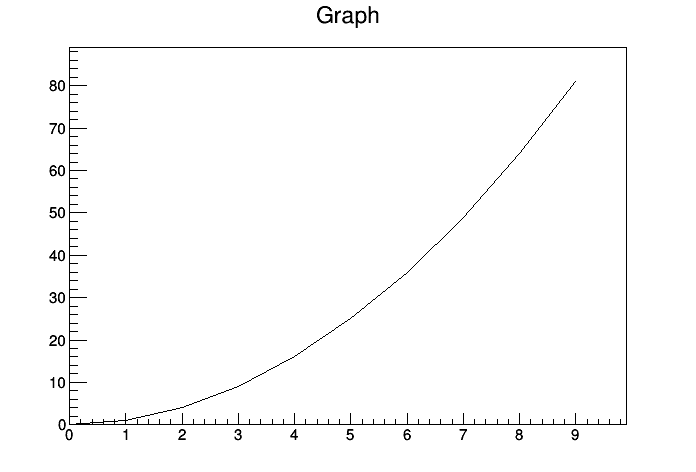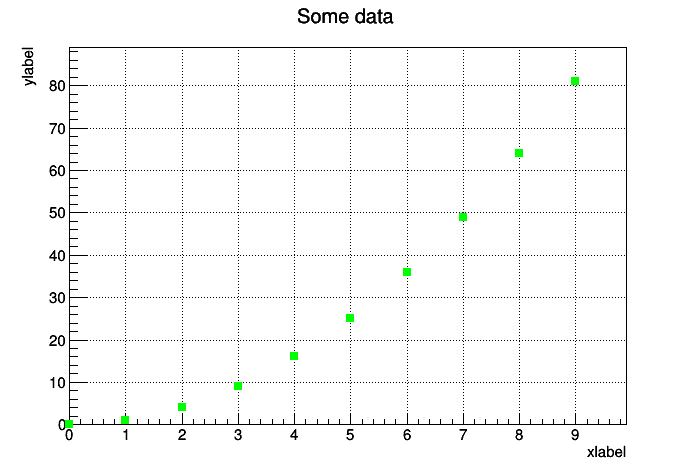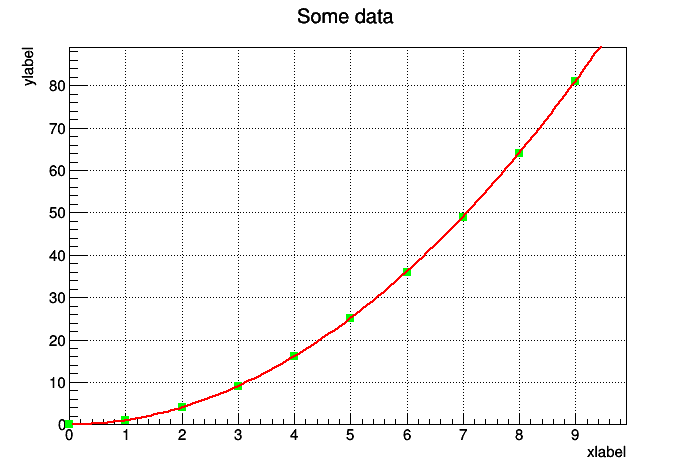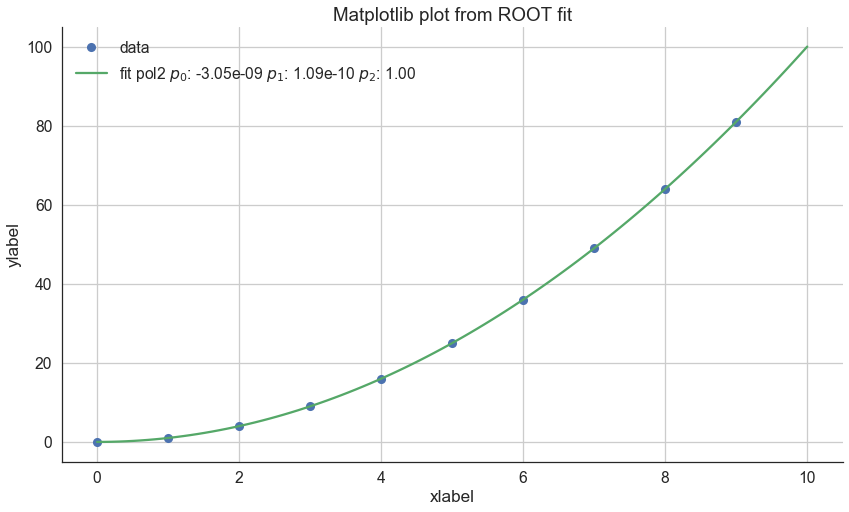# Fit data using PyROOT… and plot the result using matplotlib

ROOT is a powerful tool for data processing but the learning curve can be quite steep. Python on the other hand is in general easy to use but specially function fitting is less developed. Using a combination of ROOT trough PyROOT and Python with matplotlib and seaborn we can try to get the best of both world.

## Minimal plotting example using PyROOT

First off we start with a simple example to plot some numpy data using PyROOT. In general it is straight forward but we need to watch out with the data type that we send to the TGraph.

In :
```import numpy as np
from ROOT import TCanvas, TGraph

#Some data
x = np.arange(10)
y = x**2

#Canvas to plot on and graph
c = TCanvas()
g = TGraph(x.size, x.astype(np.double),y.astype(np.double))

#Draw canvas and graph
c.Draw()
g.Draw()
```
```Welcome to JupyROOT 6.11/01
```Next upp is adding some labels and a bit of formatting to the plot.

In :
```from ROOT import kGreen

#Formatting
g.SetLineColor( kGreen)
g.SetLineWidth( 2 )
g.SetMarkerColor( kGreen )
g.SetMarkerStyle( 21 )

#Labels and title
g.SetTitle('Some data')
g.GetXaxis().SetTitle('xlabel')
g.GetYaxis().SetTitle('ylabel')
c.SetGrid()
c.Draw()

#Draw (A)xis and (P)oints
g.Draw('AP')
```## Fit the data

ROOT has lots of build in functions but for this example we will define a custom function using the TF1 class.

In :
```#First we need a function to fit
from ROOT import TF1

#Use a custom function (altough the build in pol2 would also work)
func = TF1('func', ' + *x + *x**2', 0, 10)
fit = g.Fit('func', 'S')

c.Draw()
g.Draw('AP')
```
```****************************************
Chi2                      =  4.21991e-17
NDf                       =            7
Edm                       =  8.43981e-17
NCalls                    =           56
p0                        = -3.04736e-09   +/-   1.93046e-09
p1                        =  1.09081e-10   +/-   9.98944e-10
p2                        =            1   +/-   1.06853e-10
```## Get the fit parameters and plot in Python

Even though the ROOT offers plenty of functions to style your plots for presentations and publications I usually prefer the style from Python. Importing the seaborn package also gives you a good starting point style wise and also some extra functions for plotting.

In :
```import matplotlib.pyplot as plt
import seaborn as sns
plt.ion()

#Activate seaborn styling
sns.set()
sns.set_context('talk', font_scale = 1.2)
sns.set_style('white')

#Function to calculate y
def pol2(x, p0, p1, p2):
return p0+p1*x+p2*x**2

#Get fit parameters and generate a label
par = [fit.Get().Parameter(i) for i in range( 3 )]
l = 'fit pol2 \$p_0\$: {:.3g} \$p_1\$: {:.3g} \$p_2\$: {:.2f}'.format(*par)

#Generate an array with finer steps than x
xx = np.linspace(0, 10, 100)

#matplotlib figure and plot original and fitted data
plt.figure( figsize = (14,8) );
plt.plot(x, y, 'o', label = 'data');
plt.plot(xx, pol2(xx, *par), label = l);

#Formatting and labels
plt.grid()
plt.legend()
sns.despine()
plt.xlabel('xlabel')
plt.ylabel('ylabel')
plt.title('Matplotlib plot from ROOT fit');
```This site uses Akismet to reduce spam. Learn how your comment data is processed.